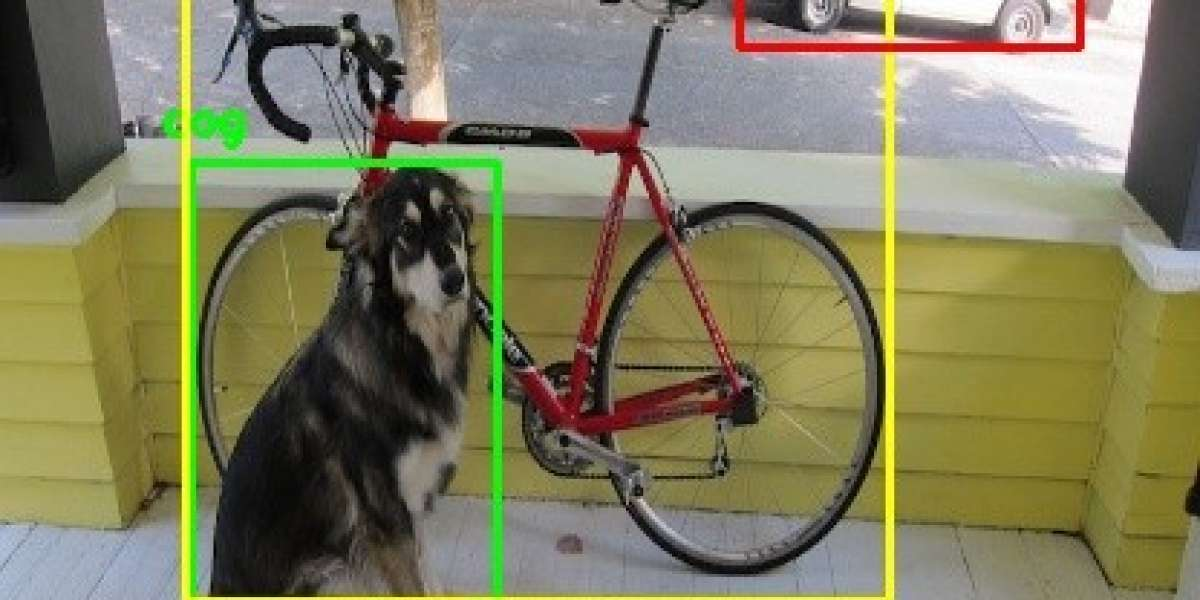## Object Detection In Computer Vision

Computer Vision field has been emerging and there are lot of improvements when it comes to detecting an object from the image and video. There are different techniques to so and here are some discussed below.

The method discussed below are in relation with the Convolutional Neural Network (CNN). Here are which I would go through:

1. R-CNN (Region Based Convolution Network)
2. Fast R-CNN
3. Faster R-CNN
4. YOLO

Region based Convolutional Neural Network (RCNN)

In RCNN, we use something called as Region Proposals. These are basically the regions that we are interested in detecting. Selective Search algorithm is used to extract these region proposals in RCNN. The algorithm in Selective Search is as follows:

1. Initial sub segmentation which would be helpful to develop the region proposals.
2. We use recurrsion in this to combine regions which are similar by looking onto neighboring regions.
3. As the final region proposals are extracted, we feed into CNN for the process like feature extraction and so on.

So, these features would be then used to classify and plot the bounding box over the object that we are interested in. The classification and bounding box can be controlled by setting a threshold value too.

RCNN was among the first used technique for object detection and it does have a number of limitations. They are:

1. Selective Search can't gurantee good region proposals and hence if this is not done nicely then the features would't be great as well, messing up our model.
2. The time taken in this process is huge as recursion is used in the process.

Fast RCNN

Fast RCNN as the name suggest is that it is process faster than traditional CNN. The changes in both the methods is that in RCNN, we feed the region proposals, unlike Fast RCNN, we give the input image directly to CNN. The selective search is still used in this process but as the image is feed and then region proposals are found in CNN, the time used for model is reduced drastically.

Faster RCNN

Faster RCNN is an improvement over Fast RCNN. We feed the image which is same as Fast RCNN. But we don't use the selective search algorithm in this. We use a separate network for the identification of the bounding box.

YOLO

This is the current state of the art model and is widely used nowadays for the object detection and creating a bounding box over the object with probability of each bounding box to give the class.

YOLO stands on You Only Look Once. As per name, we look at the image only once and this boost the process and is fastest among all the methods mentioned above.

YOLO does the classification and bounding box regression both at once unlike others.

There are various versions of YOLO which released, mostly YOLOv3 is used for the implementation now.

The algorithm is as follows:

1. The image is split into m*m grid.
2. Each grid is then taking and n bounding box are found.
3. Each bounding box is given a class and the probability for that class. We also have the threshold as a parameter which can be controlled to locate the object.

There's a limitation with YOLO that it sometimes fail to detect the small objects.

Thus, it was just a quick overview of the object detection technqiues used in Computer Vision and the comparison about the same.

Search
Categories